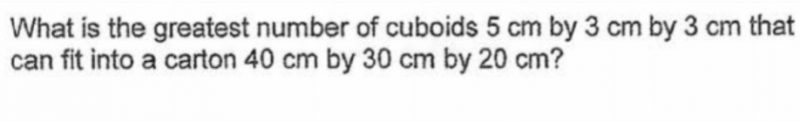# Questionhi! can help us with this qn? tq!

.

Our answer was different from the answer provided and we’re wondering how they derive at ans: 520.

Source: Methodist Girls’ School Primary

(40/5) x (30/3) x (15/3) = 400
(39/3) x (30/3) x (5/5) = 130
400 + 130 = 530

or

40/5 = 8
30/3 = 10
(20 – 5)/3 = 5 (this is to fill the carton up to 15 cm leaving 5 cm so as to fit the greatest number of cuboids into it)
8 x 10 x 5 = 400
5/5 = 1
40/3 = 13R3
30/3 = 10
1 x 13 x 10 = 130
400 + 130 = 530

Ans : 530 cuboids.

0 Replies 0 Likes